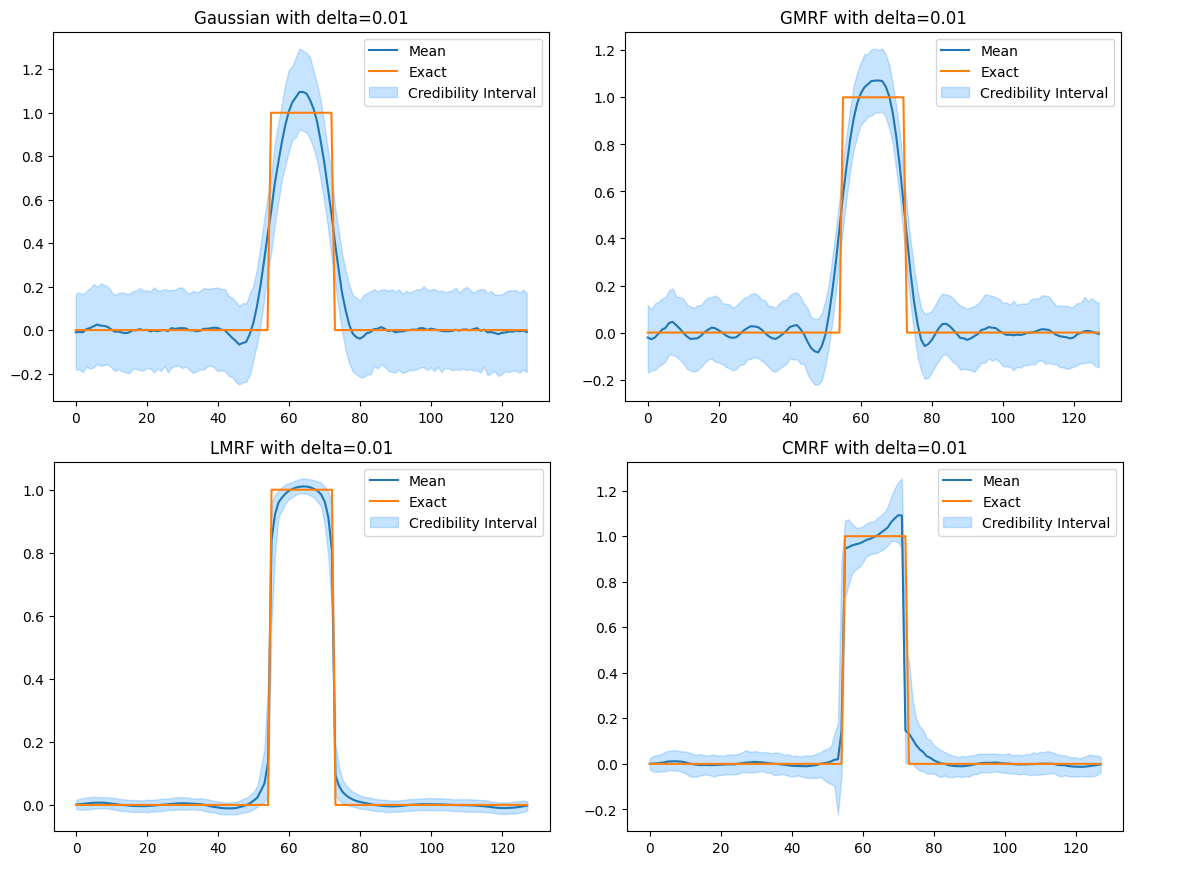# Deconvolution1D¶

## Overview¶

This benchmark is based on a 1D Deconvolution test problem from the library CUQIpy. It defines a posterior distribution for a 1D deconvolution problem, with a Gaussian likelihood and four different choices of prior distributions with configurable parameters.

Plot of data and exact solutionCredibility interval plots of posterior samples using different priors## Run¶

docker run -it -p 4243:4243 linusseelinger/benchmark-deconvolution-1d:latest


## Properties¶

Model

Description

Deconvolution1D_Gaussian

Posterior distribution with Gaussian prior

Deconvolution1D_GMRF

Posterior distribution with Gaussian Markov Random Field prior

Deconvolution1D_LMRF

Posterior distribution with Laplacian Markov Random Field prior

Deconvolution1D_CMRF

Posterior distribution with Cauchy Markov Random Field prior

Deconvolution1D_ExactSolution

Exact solution to the deconvolution problem

### Deconvolution1D_Gaussian¶

Mapping

Dimensions

Description

input



Signal $$\mathbf{x}$$

output



Log PDF $$\pi(\mathbf{b}\mid\mathbf{x})$$ of posterior with Gaussian prior

Feature

Supported

Evaluate

True

True

ApplyJacobian

False

ApplyHessian

False

Config

Type

Default

Description

delta

double

0.01

The prior parameter $$\delta$$ (see below).

### Deconvolution1D_GMRF¶

Mapping

Dimensions

Description

input



Signal $$\mathbf{x}$$

output



Log PDF $$\pi(\mathbf{b}\mid\mathbf{x})$$ of posterior with Gaussian Markov Random Field prior

Feature

Supported

Evaluate

True

True

ApplyJacobian

False

ApplyHessian

False

Config

Type

Default

Description

delta

double

0.01

The prior parameter $$\delta$$ (see below).

### Deconvolution1D_LMRF¶

Mapping

Dimensions

Description

input



Signal $$\mathbf{x}$$

output



Log PDF $$\pi(\mathbf{b}\mid\mathbf{x})$$ of posterior Laplacian Markov Random Field prior

Feature

Supported

Evaluate

True

False

ApplyJacobian

False

ApplyHessian

False

Config

Type

Default

Description

delta

double

0.01

The prior parameter $$\delta$$ (see below).

### Deconvolution1D_CMRF¶

Mapping

Dimensions

Description

input



Signal $$\mathbf{x}$$

output



Log PDF $$\pi(\mathbf{b}\mid\mathbf{x})$$ of posterior with Cauchy Markov Random Field prior

Feature

Supported

Evaluate

True

True

ApplyJacobian

False

ApplyHessian

False

Config

Type

Default

Description

delta

double

0.01

The prior parameter $$\delta$$ (see below).

### Deconvolution1D_ExactSolution¶

Mapping

Dimensions

Description

input



No input to be provided.

output



Returns the exact solution $$\mathbf{x}$$ for the deconvolution problem.

Feature

Supported

Evaluate

True

False

ApplyJacobian

False

ApplyHessian

False

Config

Type

Default

Description

None

Mount directory

Purpose

None

Sources here.

## Description¶

The 1D periodic deconvolution problem is defined by the inverse problem

$\mathbf{b} = \mathbf{A}\mathbf{x} + \mathbf{e},$

where $$\mathbf{b}$$ is an $$m$$-dimensional random vector representing the observed data, $$\mathbf{A}$$ is an $$m\times n$$ matrix representing the convolution operator, $$\mathbf{x}$$ is an $$n$$-dimensional random vector representing the unknown signal, and $$\mathbf{e}$$ is an $$m$$-dimensional random vector representing the noise.

This benchmark defines a posterior distribution over $$\mathbf{x}$$ given $$\mathbf{b}$$ as

$\pi(\mathbf{x}\mid \mathbf{b}) \propto \pi(\mathbf{b}\mid \mathbf{x})\pi(\mathbf{x}),$

where $$\pi(\mathbf{b}|\mathbf{x})$$ is a likelihood function and $$\pi(\mathbf{x})$$ is a prior distribution.

The noise is assumed to be Gaussian with a known noise level, and so the likelihood is defined via

$\mathbf{b} \mid \mathbf{x} \sim \mathcal{N}(\mathbf{A}\mathbf{x}, \sigma^2\mathbf{I}_m),$

where $$\mathbf{I}_m$$ is the $$m\times m$$ identity matrix and $$\sigma=0.01$$ defines the noise level.

The prior can be configured by choosing of the following assumptions about $$\mathbf{x}$$:

• Gaussian: Gaussian (Normal) distribution: $$x_i \sim \mathcal{N}(0, \delta)$$.

• GMRF Gaussian Markov Random Field: $$x_i-x_{i-1} \sim \mathcal{N}(0, \delta)$$.

• CMRF Cauchy Markov Random Field: $$x_i-x_{i-1} \sim \mathcal{C}(0, \delta)$$.

• LMRF Laplace Markov Random Field: $$x_i-x_{i-1} \sim \mathcal{L}(0, \delta)$$.

where $$\mathcal{C}$$ is the Cauchy distribution and $$\mathcal{L}$$ is the Laplace distribution. The parameter $$\delta$$ is the prior parameter and is configurable (see above).

The choice of prior is specified by providing the name to the HTTP model. In this case Deconvolution1D_Gaussian, Deconvolution1D_GMRF, Deconvolution1D_CMRF, and Deconvolution1D_LMRF, respectively. See um-bridge Clients for more details.

In addition to the HTTP models for the posterior, there is also an HTTP model for the exact solution to the problem. This model is called Deconvolution1D_ExactSolution and returns exact phantom used to generate the synthetic data when called.

In CUQIpy the benchmark is defined and sampled as follows:

import numpy as np
import cuqi

# Parameters
delta = 0.01
prior = "LMRF"

# Load data, define problem and sample posterior
TP = cuqi.testproblem.Deconvolution1D(
dim=128,
PSF="gauss",
PSF_param=5,
phantom="square",
noise_std=0.01,
noise_type="gaussian"
)
if prior == "Gaussian":
TP.prior = cuqi.distribution.Gaussian(np.zeros(128), delta)
elif prior == "GMRF":
TP.prior = cuqi.distribution.GMRF(np.zeros(128), 1/delta)
elif prior == "CMRF":
TP.prior = cuqi.distribution.CMRF(np.zeros(128), delta)
elif prior == "LMRF":
TP.prior = cuqi.distribution.LMRF(np.zeros(128), delta)
TP.sample_posterior(2000).plot_ci(exact=TP.exactSolution)